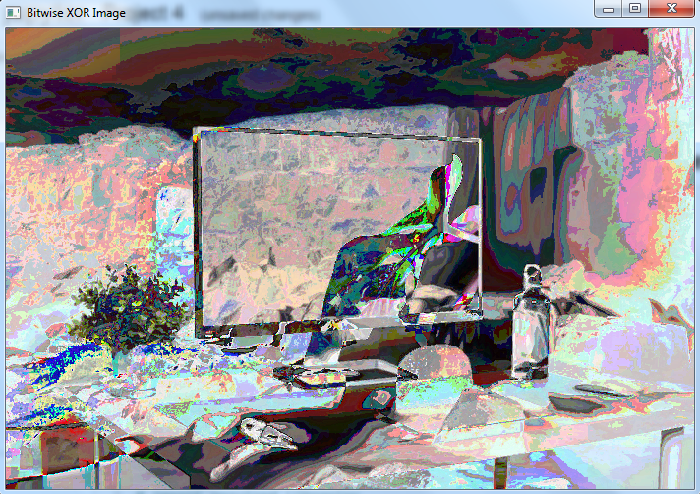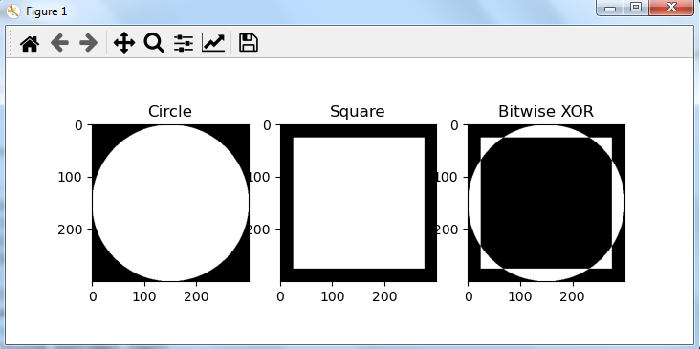# How to perform bitwise XOR operation on images in OpenCV Python?

Color images (RGB) have three channels: red, blue and green. The image is represented as a 3-dimensional numpy array. The pixel values of an image are stored using 8-bit unsigned integers (uint8) in the range "0 to 255".

The bitwise XOR operation on two images is performed on the binary representation of these pixel values of corresponding images.

Here is the syntax to perform bitwise XOR operation on two images −

cv2.bitwise_xor(img1, img2, mask=None)


Here, img1 and img2 are the two input images and mask is a mask operation.

## Steps

To compute bitwise XOR between two images, you can follow the steps given below −

Import the required libraries OpenCV, Numpy and Matplotlib. Make sure you have already installed them.

import cv2
import numpy as np
import matplotlib as plt


Read the images using cv2.imread() method. The width and height of images must be the same.

img1 = cv2.imread('waterfall.jpg')


Compute the bitwise XOR on two images using cv2.biwise_xor(img1, img2).

xor_img = cv2.bitwise_xor(img1,img2)


Display the bitwise XOR image.

cv2.imshow('Bitwise XOR Image', xor_img)
cv2.waitKey(0)
cv2.destroyAllWindows()


We will use the following images as the Input Files in the examples below.## Example 1

In the Python program below, we compute the bitwise XOR on the two color images.

# import required libraries
import cv2

# Read two images. The size of both images must be the same.

# compute bitwise XOR on both images
xor_img = cv2.bitwise_xor(img1,img2)

# display the computed bitwise XOR image
cv2.imshow('Bitwise XOR Image', xor_img)
cv2.waitKey(0)
cv2.destroyAllWindows()


## Output

When you run this Python program, it will produce the following output −## Example 2

The following program shows the application of bitwise XOR operation on two images. We created two images, first a circle and second a square of the same size.

# import required libraries
import cv2
import numpy as np
import matplotlib.pyplot as plt

# define first image as a circle
img1 = np.zeros((300, 300), dtype = "uint8")
img1 = cv2.circle(img1, (150, 150), 150, 255, -1)

# define second image as a square
img2 = np.zeros((300,300), dtype="uint8")
img2 = cv2.rectangle(img2, (25, 25), (275, 275), 255, -1)

# perform bitwise XOR on img1 and img2
xor_img = cv2.bitwise_xor(img1,img2)

# Display the bitwise XOR output image
plt.subplot(131), plt.imshow(img1, 'gray'), plt.title("Circle")
plt.subplot(132), plt.imshow(img2,'gray'), plt.title("Square")
plt.subplot(133), plt.imshow(xor_img, 'gray'), plt.title("Bitwise XOR")

plt.show()


## Output

When you run this Python program, it will produce the following output